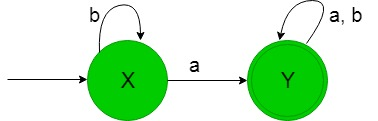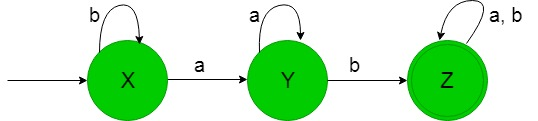# Designing Deterministic Finite Automata (Set 3)

Prerequisite: Designing finite automata, Designing Deterministic Finite Automata (Set 2)
In this article, we will see some designing of Deterministic Finite Automata (DFA).

Problem-1: Construction of a minimal DFA accepting set of string over {a, b} where each string containing ‘a’ as the substring.

Explanation:
The desired language will be like:

`L1 = {a, aa, ab, ba, ..............}`

Here as we can see that each string of the language containing ‘a’ as the substring but the below language is not accepted by this DFA because some of the string of the below language does not contain ‘a’ as the substring.

`L2 = {ba, bb, bbb, ..............}`

The state transition diagram of the language containing ‘a’ as the substring will be like:In the above DFA, states ‘X’ and ‘Y’ are the initial and final state respectively, it accepts all the strings containing ‘a’ as the substring. Here as we see that on getting input as ‘b’ it remains in the state of ‘X’ itself but on getting ‘a’ as the input it transit to final state ‘Y’ and hence such string is accepted by above DFA.

Problem-2: Construction of a minimal DFA accepting set of string over {a, b} where each string containing ‘ab’ as the substring.

Explanation: The desired language will be like:

`L1 = {ab, aab, abb, bab, ..............}`

Here as we can see that each string of the language containing ‘ab’ as the substring but the below language is not accepted by this DFA because some of the string of the below language does not contain ‘ab’ as the substring-

`L2 = {aba, bba, bbbaaa, ..............}`

The state transition diagram of the language containing ‘ab’ as the substring will be like:In the above DFA, states ‘X’ and ‘Z’ are the initial and final state respectively, it accepts all the strings containing ‘ab’ as the substring. Here as we see that on getting ‘b’ as the input it remains in the state of initial state itself, on getting ‘a’ as the input it transit to state ‘Y’ and then on getting ‘b’ it finally transit to the final state ‘Z’ and hence this DFA is accepting all the language containing ‘ab’ as the substring.My Personal Notes arrow_drop_upCheck out this Author's contributed articles.

If you like GeeksforGeeks and would like to contribute, you can also write an article using contribute.geeksforgeeks.org or mail your article to contribute@geeksforgeeks.org. See your article appearing on the GeeksforGeeks main page and help other Geeks.

Please Improve this article if you find anything incorrect by clicking on the "Improve Article" button below.